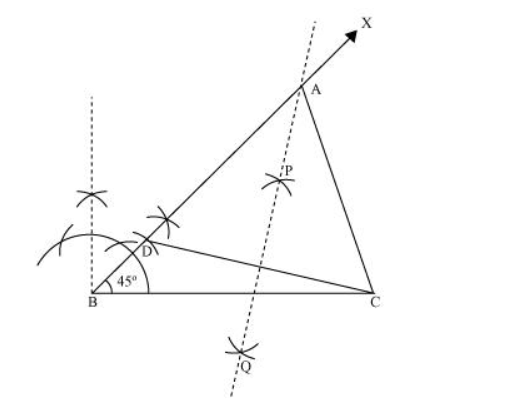# Construct a triangle ABC in which BC = 8 cm,Question. Construct a triangle $A B C$ in which $B C=8 \mathrm{~cm}, \angle B=45^{\circ}$ and $A B-A C=3.5 \mathrm{~cm}$.

Solution:

The below given steps will be followed to draw the required triangle.

Step I: Draw the line segment $B C=8 \mathrm{~cm}$ and at point $B$, make an angle of $45^{\circ}$, say $\angle X B C$.

Step II: Cut the line segment $B D=3.5 \mathrm{~cm}$ (equal to $A B-A C$ ) on ray $B X$.

Step III: Join DC and draw the perpendicular bisector PQ of DC.

Step IV: Let it intersect $B X$ at point $A$. Join $A C . \triangle A B C$ is the required triangle.